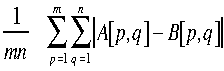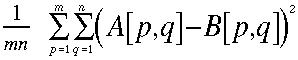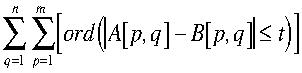## Matching Criteria for BMAs

A matching criterion, or distortion function, is used to quantify the similarity between the target block and candidate blocks. A number of matching criteria are suitable for use in video compression.

The mean absolute difference (MAD) is the most popular block matching criterion. Corresponding pixels from each block are compared and their differences summed, as described by this equation.Mean Absolute Difference function for two blocks A and B of size nxm. A[p,q] is the value of the pixel in the pth row and qth column of block A.

The lower the MAD the better the match and so the candidate block with the minimum MAD should be chosen. The function is alternatively called Mean Absolute Error (MAE).
The MAD criterion has been used by many authors [Jaur90] [Lin84] [Lall91] [DelR90].

### Mean Square Difference (MSD)

The mean square difference function is similar to the mean absolute difference function, except that the difference between pixels is squared before summation.Mean Squared Difference function

The mean square difference is more commonly called the mean square error (MSE) and the lower this value the better the match. This criterion is said to result in better matches than the MAD criterion [Ahma90] although Dianysian and Baker found the difference between it and Mean Square Difference (MSD) criterion was too slight to be perceived [Dian88].

### Pel Difference Classification (PDC)

The Pel Difference Classification (PDC) distortion function compares each pixel of the target block with its counterpart in the candidate block and classifies each pixel pair as either matching or not matching. Pixels are matching if the difference between their values is less than some threshold and the greater the number of matching pixels the better the match.Pel Difference Classification function. Ord(e) evaluates to 1 if e is true and false otherwise.

Chan et al. used a modified form of the PDC in which blocks were ruled out of the search by varying the threshold, beginning with an arbitrarily high threshold and halving it until only a few candidate blocks remained. [Chan94]

### Integral Projection

In 1992 Kim and Park proposed using integral projections (IP) as a matching criterion [Kim92]. Integral projections are calculated by summing the values of pixels from each column and each row of a block. The most attractive feature of this criterion is that values calculated for a particular candidate block can be reused in calculating the integrals for overlapping candidate blocks. This feature is of particular value during an exhaustive search, but less useful in the case of sub-optimal searches. Kim and Park claimed that this choice of matching criterion is less susceptible to noise than others.Integral Projection functionIntegral Projection function